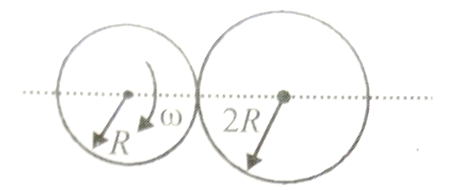# NEET Physics Mathematical Tools Questions Solved

Two discs of radii R and 2R are pressed against each other. Initially disc with radius R is rotating with angular velocity $\omega$ and other disc was stationary. Both disc are hinged at their respective centres and free to rotate about them.Moment of inertia of smaller is I and bigger disc is 2I about their respective axis of rotation. Find the angular velocity of the bigger disc after long time.

[This question is only for Dropper and XII batch]1. $\omega$

2. $\omega$/2

3. $\omega$/3

4. $\frac{2\omega }{3}$